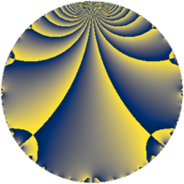# Properties

 Label 784.2.a.bLevel $784$ Weight $2$ Character orbit 784.a Self dual yes Analytic conductor $6.260$ Analytic rank $1$ Dimension $1$ CM no Inner twists $1$

# Related objects

## Newspace parameters

 Level: $$N$$ $$=$$ $$784 = 2^{4} \cdot 7^{2}$$ Weight: $$k$$ $$=$$ $$2$$ Character orbit: $$[\chi]$$ $$=$$ 784.a (trivial)

## Newform invariants

 Self dual: yes Analytic conductor: $$6.26027151847$$ Analytic rank: $$1$$ Dimension: $$1$$ Coefficient field: $$\mathbb{Q}$$ Coefficient ring: $$\mathbb{Z}$$ Coefficient ring index: $$1$$ Twist minimal: no (minimal twist has level 14) Fricke sign: $$1$$ Sato-Tate group: $\mathrm{SU}(2)$

## $q$-expansion

 $$f(q)$$ $$=$$ $$q - 2q^{3} + q^{9} + O(q^{10})$$ $$q - 2q^{3} + q^{9} + 4q^{13} - 6q^{17} + 2q^{19} - 5q^{25} + 4q^{27} - 6q^{29} - 4q^{31} + 2q^{37} - 8q^{39} - 6q^{41} - 8q^{43} - 12q^{47} + 12q^{51} + 6q^{53} - 4q^{57} - 6q^{59} - 8q^{61} + 4q^{67} - 2q^{73} + 10q^{75} - 8q^{79} - 11q^{81} - 6q^{83} + 12q^{87} + 6q^{89} + 8q^{93} + 10q^{97} + O(q^{100})$$

## Embeddings

For each embedding $$\iota_m$$ of the coefficient field, the values $$\iota_m(a_n)$$ are shown below.

For more information on an embedded modular form you can click on its label.

Label $$\iota_m(\nu)$$ $$a_{2}$$ $$a_{3}$$ $$a_{4}$$ $$a_{5}$$ $$a_{6}$$ $$a_{7}$$ $$a_{8}$$ $$a_{9}$$ $$a_{10}$$
1.1
 0
0 −2.00000 0 0 0 0 0 1.00000 0
 $$n$$: e.g. 2-40 or 990-1000 Significant digits: Format: Complex embeddings Normalized embeddings Satake parameters Satake angles

## Atkin-Lehner signs

$$p$$ Sign
$$2$$ $$-1$$
$$7$$ $$-1$$

## Inner twists

This newform does not admit any (nontrivial) inner twists.

## Twists

By twisting character orbit
Char Parity Ord Mult Type Twist Min Dim
1.a even 1 1 trivial 784.2.a.b 1
3.b odd 2 1 7056.2.a.bd 1
4.b odd 2 1 98.2.a.a 1
7.b odd 2 1 112.2.a.c 1
7.c even 3 2 784.2.i.i 2
7.d odd 6 2 784.2.i.c 2
8.b even 2 1 3136.2.a.z 1
8.d odd 2 1 3136.2.a.e 1
12.b even 2 1 882.2.a.i 1
20.d odd 2 1 2450.2.a.t 1
20.e even 4 2 2450.2.c.c 2
21.c even 2 1 1008.2.a.h 1
28.d even 2 1 14.2.a.a 1
28.f even 6 2 98.2.c.b 2
28.g odd 6 2 98.2.c.a 2
35.c odd 2 1 2800.2.a.g 1
35.f even 4 2 2800.2.g.h 2
56.e even 2 1 448.2.a.g 1
56.h odd 2 1 448.2.a.a 1
84.h odd 2 1 126.2.a.b 1
84.j odd 6 2 882.2.g.c 2
84.n even 6 2 882.2.g.d 2
112.j even 4 2 1792.2.b.c 2
112.l odd 4 2 1792.2.b.g 2
140.c even 2 1 350.2.a.f 1
140.j odd 4 2 350.2.c.d 2
168.e odd 2 1 4032.2.a.w 1
168.i even 2 1 4032.2.a.r 1
252.s odd 6 2 1134.2.f.f 2
252.bi even 6 2 1134.2.f.l 2
308.g odd 2 1 1694.2.a.e 1
364.h even 2 1 2366.2.a.j 1
364.p odd 4 2 2366.2.d.b 2
420.o odd 2 1 3150.2.a.i 1
420.w even 4 2 3150.2.g.j 2
476.e even 2 1 4046.2.a.f 1
532.b odd 2 1 5054.2.a.c 1
644.h odd 2 1 7406.2.a.a 1

By twisted newform orbit
Twist Min Dim Char Parity Ord Mult Type
14.2.a.a 1 28.d even 2 1
98.2.a.a 1 4.b odd 2 1
98.2.c.a 2 28.g odd 6 2
98.2.c.b 2 28.f even 6 2
112.2.a.c 1 7.b odd 2 1
126.2.a.b 1 84.h odd 2 1
350.2.a.f 1 140.c even 2 1
350.2.c.d 2 140.j odd 4 2
448.2.a.a 1 56.h odd 2 1
448.2.a.g 1 56.e even 2 1
784.2.a.b 1 1.a even 1 1 trivial
784.2.i.c 2 7.d odd 6 2
784.2.i.i 2 7.c even 3 2
882.2.a.i 1 12.b even 2 1
882.2.g.c 2 84.j odd 6 2
882.2.g.d 2 84.n even 6 2
1008.2.a.h 1 21.c even 2 1
1134.2.f.f 2 252.s odd 6 2
1134.2.f.l 2 252.bi even 6 2
1694.2.a.e 1 308.g odd 2 1
1792.2.b.c 2 112.j even 4 2
1792.2.b.g 2 112.l odd 4 2
2366.2.a.j 1 364.h even 2 1
2366.2.d.b 2 364.p odd 4 2
2450.2.a.t 1 20.d odd 2 1
2450.2.c.c 2 20.e even 4 2
2800.2.a.g 1 35.c odd 2 1
2800.2.g.h 2 35.f even 4 2
3136.2.a.e 1 8.d odd 2 1
3136.2.a.z 1 8.b even 2 1
3150.2.a.i 1 420.o odd 2 1
3150.2.g.j 2 420.w even 4 2
4032.2.a.r 1 168.i even 2 1
4032.2.a.w 1 168.e odd 2 1
4046.2.a.f 1 476.e even 2 1
5054.2.a.c 1 532.b odd 2 1
7056.2.a.bd 1 3.b odd 2 1
7406.2.a.a 1 644.h odd 2 1

## Hecke kernels

This newform subspace can be constructed as the intersection of the kernels of the following linear operators acting on $$S_{2}^{\mathrm{new}}(\Gamma_0(784))$$:

 $$T_{3} + 2$$ $$T_{5}$$ $$T_{11}$$

## Hecke characteristic polynomials

$p$ $F_p(T)$
$2$ $$T$$
$3$ $$2 + T$$
$5$ $$T$$
$7$ $$T$$
$11$ $$T$$
$13$ $$-4 + T$$
$17$ $$6 + T$$
$19$ $$-2 + T$$
$23$ $$T$$
$29$ $$6 + T$$
$31$ $$4 + T$$
$37$ $$-2 + T$$
$41$ $$6 + T$$
$43$ $$8 + T$$
$47$ $$12 + T$$
$53$ $$-6 + T$$
$59$ $$6 + T$$
$61$ $$8 + T$$
$67$ $$-4 + T$$
$71$ $$T$$
$73$ $$2 + T$$
$79$ $$8 + T$$
$83$ $$6 + T$$
$89$ $$-6 + T$$
$97$ $$-10 + T$$